# Online Civil Engineering Test - Surveying TestLoading Test...

Instruction:

• This is a FREE online test. DO NOT pay money to anyone to attend this test.
• Total number of questions : 20.
• Time alloted : 30 minutes.
• Each question carry 1 mark, no negative marks.
• DO NOT refresh the page.
• All the best :-).

1.

If θ is the slope of the ground and l is the measured distance, the correction is

A.
 2l sin2 θ/2B.
 2l cos2 θ/2C.
 2l tan2 θ/2D.
 2l cot2 θ/2.2.

Let angular value of one graduation of a tube of length x be φ seconds and R be the radius of its internal curved surface, then

A.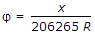B.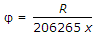C.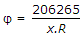D.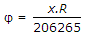.3.

In an internal focusing telescope

A.
 the objective is at a fixed distance from the diaphragmB.
 the focusing is done by the sliding of a divergent lens.C.
 the focusing divergent lens is situated at about the middle of the tubeD.
 all the above.4.

The Random errors tend to accumulate proportionally to

A.
 numbers of operations involvedB.
 reciprocal of operations involvedC.
 square root of the number of operation involvedD.
 cube root of the number of operation involved.5.

A clinometer is used for

A.
 measuring angle of slopeB.
 correcting line of collimationC.
 setting out right anglesD.
 defining natural features.6.

The 'fix' of a plane table from three known points, is good, if

A.
 middle station is nearestB.
 middle station is farthestC.
 either the right or left station is nearestD.
 none of these.7.

For orientation of a plane table with three points A, B and C, Bessel's drill is

A.
 Align b through a and draw a ray towards c, align a through b and draw a ray towards c, finally align c through the point of intersection of the previously drawn raysB.
 Align c through a and draw a ray towards b, align a through c and draw a ray towards b, finally align b through the point of intersection of the previously drawn raysC.
 Align c through b and draw a ray towards a, align b through c and draw a ray towards a, finally align a, through the point of intersection of the previously, drawn raysD.
 In the first two steps any two of the points may be used and a ray drawn towards the third point, which is sighted through the point of intersection of previously drawn rays in the final step.8.

If Δ is the angle of deflection of the curve, T1 and T2 are its points of tangencies, the angle between the tangent at T1 and long chord T1 T2 will be

A.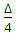B.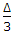C.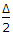D.
 ΔE.
 2 Δ.9.

The method of finding out the difference in elevation between two points for eliminating the effect of curvature and refraction, is

A.
 reciprocal levellingB.
 precise levellingC.
 differential levellingD.
 flying levelling10.

The bearings of two traverse legs AB and BC are N52° 45' E and N34° 30' E respectively. The deflection angle is

A.
 18° 15' EB.
 18° 15' NC.
 18° 15' WD.
 18° 15' RE.
 18° 15' L11.

Greater accuracy in linear measurements, is obtained by

A.
 tacheometryB.
 direct chainingC.
 direct tapingD.
 all the above.12.

The bearing of AB is 190° and that of CB is 260° 30'. The included angle ABC, is

A.
 80° 30'B.
 99° 30'C.
 70° 30'D.
 none of these13.

The ratio of the distances at which a stated length can be distinguished by the telescope and the human eye, respectively, is called

A.
 brightness of telescopeB.
 magnification of telescopeC.
 resolving power of telescopeD.
 none of these.14.

Check lines (or proof lines) in Chain Surveying, are essentially required

A.
 to plot the chain linesB.
 to plot the offsetsC.
 to indicate the accuracy of the survey workD.
 to increase the out-turn15.

The Huygen's telescope eye piece

A.
 is aplanaticB.
 achromaticC.
 both (a) and (b)D.
 neither (a) nor (b).16.

Pick up the correct statement from the following :

A.
 it is difficult to eliminate an error completely at first trialB.
 instability of the instrument makes it almost impossible to adjust it satisfactorilyC.
 adjustment screws must be left bearing firmly but should never be forcedD.
 all the above.17.

Surveys which are carried out to provide a national grid of control for preparation of accurate maps of large areas, are known

A.
 plane surveysB.
 geodetic surveysC.
 geographical surveysD.
 topographical surveys.18.

The 10 mm markings on a levelling staff placed at 20 m are separated by

A.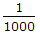radianB.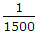radianC.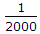radianD.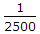radian.19.

The additional lines which are measured to show the correctness of the chain surveying are called:

A.
 check clinesB.
 proof linesC.
 tie linesD.
 all of these.20.

If D is the degree of the curve of radius R, the exact length of its specified chord, is

A.
 radius of the curve x sine of half the degreeB.
 diameter of the curve x sine of half the degreeC.
 diameter of the curve x cosine of half the degreeD.
 diameter of the curve x tangent of half the degree.